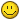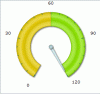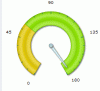# darry chui

Members

3

## Posts posted by darry chui

1. ### Day Chart

I am in need to create a rolling day chart. How can I represent a rolling 30 day chart and color code per day either red or green?

Any help would be appreciated.

Thanks

2. ### Upper Limit And Dial Value

Hi,

Welcome to FusionCharts Forum!Here is my xml:

<Chart palette="3" bgColor="FFFFFF" bgAlpha="100" lowerLimit="0" upperLimit="120" gaugeStartAngle="240" gaugeEndAngle="-60" gaugeInnerRadius="60%" showToolTip="0" gaugeFillMix="{light-10},{light-30},{light-20},{dark-5},{color},{light-30},{light-20},{dark-10}" gaugeFillRatio="" baseFontColor="000000" valueBelowPivot="1" decimals="1">

<colorRange>

<color minValue="0" maxValue="60" code="e1c705"/>

<color minValue="60" maxValue="120" code="8ce105"/>

</colorRange>

<dials>

<dial id="Dial1" value="3" baseWidth="6" topWidth="1" editMode="0"/>

</dials>

<styles>

<definition>

<style type="font" name="valueFont" bold="1" size="8" color="0085CF"/>

</definition>

<application>

<apply toObject="value" styles="valueFont"/>

</application>

</styles>

</Chart>

As I stated before, I want to limit the upper bound to 120 but display the true value in the center.

TIA3. ### Upper Limit And Dial Value

Hi, I am working on the Angular Gauge with a lower limit of 0 and a upper limit of 120.

What I want to do is when the value is above 120 to have the dial peg at 120, but the display value at the center of the dial to display the true value.

Right now what is does is the upper limit on the chart gets pushed out.

Let me know if this can be done.

Thanks